MORE IN Analog Electronics - 1
MU Electronics and Telecom Engineering (Semester 3)
Analog Electronics - 1
December 2013
Total marks: --
Total time: --
INSTRUCTIONS
(1) Assume appropriate data and state your reasons
(2) Marks are given to the right of every question
(3) Draw neat diagrams wherever necessary

Solve any five:-
1 (a) Draw switching characteristics of a diode and explain reverse recovery time
4 M
1 (b) Draw energy bond diagram of MOS capacitor in accumulation, depletion and inversion region for P-substrate
4 M
1 (c) Draw the dc load line for above circuit.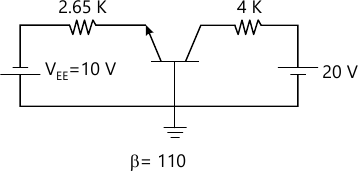4 M
1 (d) Compare CE, CB and CC configuration
4 M
1 (e) Obtain gm, ro and Av for the amplifier circuit shown in figure. In which region the device is operating? Justify.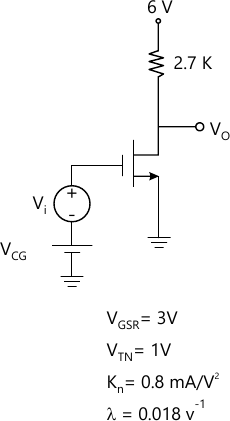4 M
1 (f) State and explain Barkhausen's Criteria for oscillation
4 M

2 (a) Obtain IDQ, VDSQ, VGSQ graphically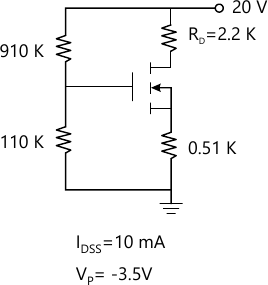8 M
2 (b) Derive the expression for frequency of oscillation for a transistorized (BJT) RC phase shift oscillator
8 M
2 (c) Obtain output for the clipper circuit shown in fig. If a sine wave of 15 sinwt is applied as an input. Assume practicle diode with suitable cut in voltage.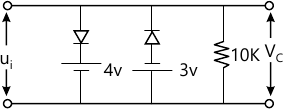4 M

3 (a) VTN=1V, Kn=05 mA/V2. ?=0.01 V-1 Determine VGSQ and VDSQ Also calculate voltage gain, input and output resistance.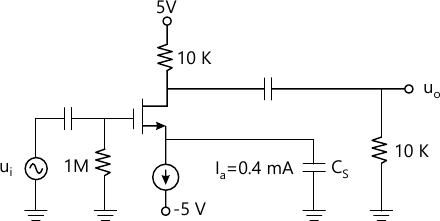10 M
3 (b) Determine Ri, Ro, Av and gm for amplifier circuit shown in figure VBE(ON)=0.7V, β=100, VA=∞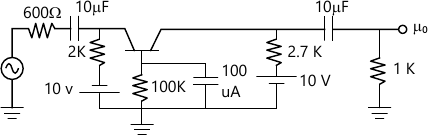10 M

4 (a) Derive the expression for Threshold Voltage for Enhancement type N-channel MOSFET
10 M
4 (b) Determine IB, ICE, VE and VB and also SICO for the biasing circuit shown in figure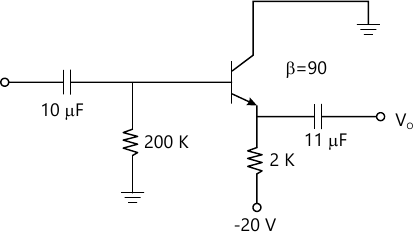10 M

5 (a) Explain graphical method to obtain to parameter of CE configuration
10 M
5 (b) Calculate IDQ, VGSQ and VDSQ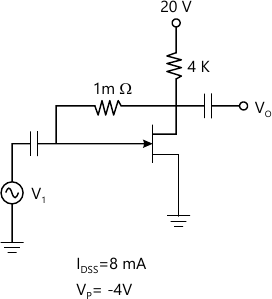5 M
5 (c) Determine hybrid-? parameters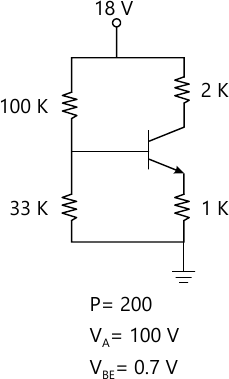5 M

Write short note (any four) :-
6 (a) Small signal model of a diode
5 M
6 (b) Hybrid pi model of BJT
5 M
6 (c) Regions of operation of FET
5 M
6 (d) Crystal oscilator
5 M
6 (e) Construction and operation of schottkey diode
5 M

More question papers from Analog Electronics - 1